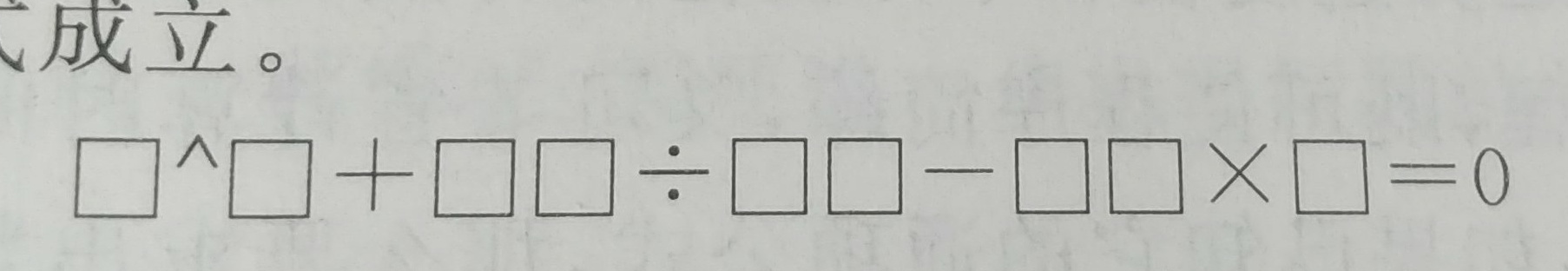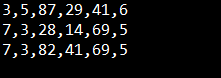算法学习——枚举之完美综合式

算法描述1. 各个一位数不能是1
2. 只能填写1~9的数字
3. 方框里的数字不能重复

算法思路

1. 首先是将每个一位数和两位数都看成一个数，我们可以使用a,b,c,d,e,f六个数代替

2. 设置for循环，枚举a,b,c,d,e,f这六个数

a 范围2~9（因为是不允许各个一位数为1）

b 范围2~9（同理）

c 范围10~99

d 范围10~99

e 范围10~99

f 范围2~9

这里需要注意的是c和d的枚举，设置的时候有些巧妙，由于d是被除数，所以我们优先改变的应该是c,而且，由题目我们可以猜测出，这里的c和d应该是可以整除的，所以我们可以将c作为d的倍数增加

for(d=10;d<=99;d++)

for(c=d;c<=99;c=c+d)

3. 乘方函数可以使用java中Math.pow函数

4. 设置一个函数判断式子是否成立，成立则返回true，不成立则返回false

5. 设置一个函数判断式子中的各个数是否包含0，是否重复，返回类型也是一个boolean类型

6. 上述两个函数返回的数值均是true则可以判断a,b,c,d,e,f是满足要求的数字，打印出来（或者是使用整除和取余的方法一个个数的取出）

算法实现

int a,b,c,d,e,f;
for(a=2;a<=9;a++){
for(b=2;b<=9;b++){
for(d=10;d<=99;d++){
for(c=d;c<=99;c=c+d){
for(e=10;e<=99;e++){
for(f=2;f<=9;f++){
if(jisuan(a, b, c, d, e, f)){
if(isChongfu(a, b, c, d, e, f)){
System.out.println(a+","+b+","+c+","+d+","+e+","+f);
}
}
}
}
}
}
}
}
}
public static boolean jisuan(int a,int b,int c,int d,int e,int f){
return (Math.pow(a, b) + c/d )==e*f;
}
public static boolean isChongfu(int a,int b,int c,int d,int e,int f){
int[] flag = new int;
flag=a;
flag=b;
flag=c/10;
flag=c%10;
flag=d/10;
flag=d%10;
flag=e/10;
flag=e%10;
flag=f;
for(int i=0;i<flag.length;i++){
if(flag[i]==0){
return false;
}
}
for(int i=0;i<flag.length-1;i++){
for(int j=i+1;j<flag.length;j++){
if(flag[i]==flag[j]){
return false;
}
}
}
return true;
}

结果posted @ 2018-09-24 16:00  Stars-one  阅读(841)  评论(0编辑  收藏  举报